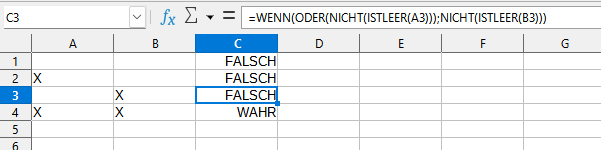# Conditions if/or: Bug or human error?

Hello!

Not sure if I am doing something wrong, or if I hit a bug: With (the german versions of) LO Calc 7.5.1.2 and 7.4.6.2, `=IF(OR(NOT(ISEMPTY(A1)));NOT(ISEMPTY(A2)))` returns `TRUE` only when `A1` AND `A2` are not empty, just as `=IF(AND(NOT(ISEMPTY(A1)));NOT(ISEMPTY(A2)))`.Please clarify - do you need to compare cells in a column, one under the other, as it is written in the formulas in the text of the question, or two adjacent cells in a row, as in the screenshot?

1 Like

An unrelated question: why do you use the `IF` here? `=OR(NOT(ISBLANK(A1));NOT(ISBLANK(A2)))` will give you the result.

1 Like

…or just `=AND((A3<>"");(B3<>""))`?

@mikekaganski mikekaganski:
Thank you, solved!

@JohnSUN: Sorry, translation error!

Seeing that the answer from @mikekaganski was what you were after (which I also thought you had meant), the cause of your seemingly faulty formula result is a mistake in bracketing. IOW, human error, not a bug.

You wrote `=WENN(ODER(NICHT(ISTLEER(A3)));NICHT(ISTLEER(B3)))`

Here, the OR function call (ODER) is closed after the first argument. The second `NICHT(ISTLEER(...))` becomes the return value of the IF function (WENN) instead of the last OR-ed condition.

You meant `=WENN(ODER(NICHT(ISTLEER(A3));NICHT(ISTLEER(B3))))`

1 Like